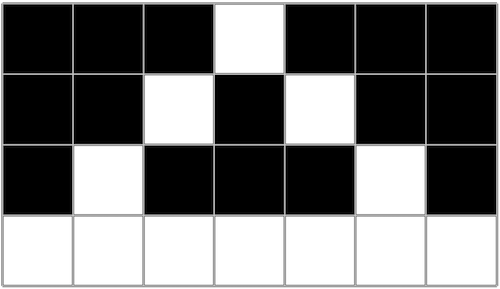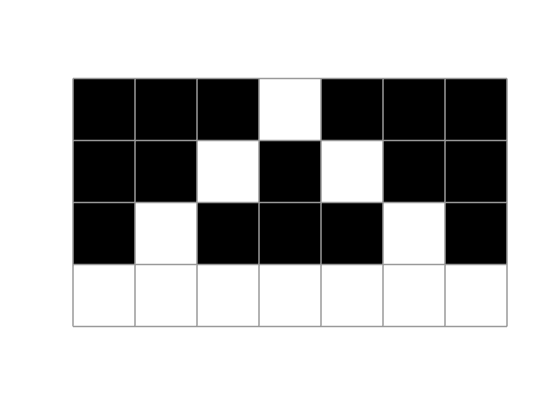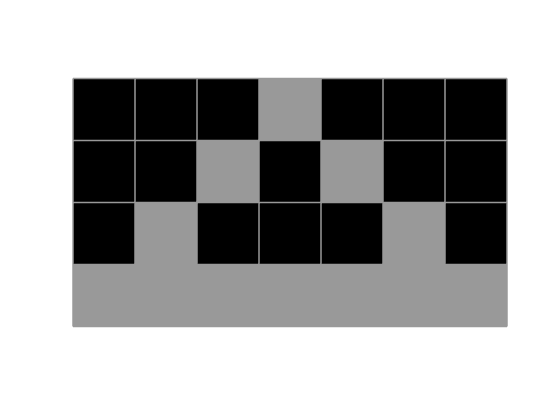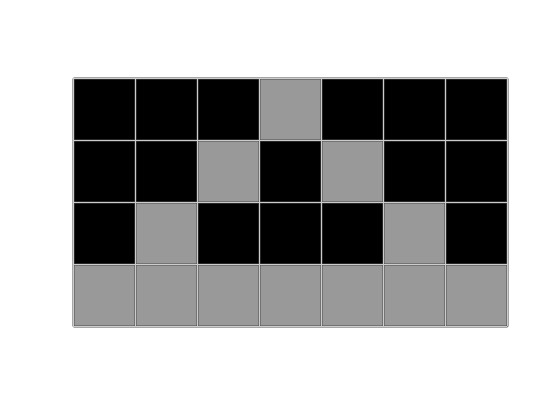# pixelgrid

### Contents

#### Introduction

Some years ago, I blogged about how to overlay a pixel grid on an image, so that you could clearly see pixel boundaries on a zoomed-in view of the image. Something like this:I intended to submit the code to the File Exchange, but I got sidetracked and never did that. But a reader asked me about it recently, so I found the code and finally submitted it.

Here's the code for making the tiny image (only 4x7) shown above.

bw = [...
0 0 0 1 0 0 0
0 0 1 0 1 0 0
0 1 0 0 0 1 0
1 1 1 1 1 1 1 ]

bw =

0     0     0     1     0     0     0
0     0     1     0     1     0     0
0     1     0     0     0     1     0
1     1     1     1     1     1     1



And here's what it looks like when you display it using imshow.

imshow(bw,'InitialMagnification','fit')It's hard to see the individual pixels, and there's a whole row of white pixels at the bottom of the image that have disappeared into the background of the page. It would be nice to have a subtle but visible outline around each pixel. Here's some basic code that draws some gray lines on top of the lines.

hold on
for x = 0.5:1:7.5
xx = [x x];
yy = [0.5 4.5];
plot(xx,yy,'Color',[0.6 0.6 0.6]);
end

for y = 0.5:1:4.5
xx = [0.5 7.5];
yy = [y y];
plot(xx,yy,'Color',[0.6 0.6 0.6]);
end
hold offThat works for the image above, but it won't work in general. No matter what color we choose for the pixel outlines, there is always the possibility that some image pixels will be the same or close to that color. For example:

f = bw;
f(f ~= 0) = 0.6;
imshow(f,'InitialMagnification','fit')

hold on
for x = 0.5:1:7.5
xx = [x x];
yy = [0.5 4.5];
plot(xx,yy,'Color',[0.6 0.6 0.6]);
end

for y = 0.5:1:4.5
xx = [0.5 7.5];
yy = [y y];
plot(xx,yy,'Color',[0.6 0.6 0.6]);
end
hold offTo solve this problem, I take the approach of drawing two different lines, in the same locations, with different thicknesses and contrasting colors. That way, the outline is guaranteed to always be visible, regardless of the underlying image pixel colors.

Here are the basic steps followed by my pixelgrid function on the File Exchange:

1. Find the image object.
2. Get the spatial coordinates for the left, right, top, and bottom of every row and column of image pixels.
3. Construct a single x-vector and a single y-vector that can be used to draw the entire outline.
4. Draw the outline using two contrasting lines.

Here's a new figure with an image displayed so that I can show how each step works.

figure
imshow(f,'InitialMagnification','fit')#### Find the image object

I want to be able to call pixelgrid with no arguments and just it find everything it needs automatically.)

h_im = findobj(gca,'type','image');


#### Get the spatial coordinates for drawing the outline

The image object has this information. It can tell us the number of rows and columns in the image, as well as the spatial coordinates for the left, right, top, and bottom edges of the image.

[M,N,~] = size(h_im.CData);
xdata = h_im.XData;
ydata = h_im.YData;

pixel_height = (ydata(2) - ydata(1)) / (M-1)

pixel_height =

1


pixel_width = (xdata(2) - xdata(1)) / (N-1)

pixel_width =

1



(Note: pixelgrid contains additional code to handle the special case where the image has only one row or column.)

#### Construct the x- and y-vectors

Pay attention to the need to draw the outlines in between the pixel centers.

y_top = ydata(1) - (pixel_height/2);
y_bottom = ydata(2) + (pixel_height/2);
y = linspace(y_top, y_bottom, M+1);

x_left = xdata(1) - (pixel_width/2);
x_right = xdata(2) + (pixel_width/2);
x = linspace(x_left, x_right, N+1);


xv1 and yv1 are used to draw vertical line segments. The coordinates of each vertical segment are separated by NaNs.

xv1 = NaN(1,3*numel(x));
xv1(1:3:end) = x;
xv1(2:3:end) = x;
yv1 = repmat([y(1) y(end) NaN], 1, numel(x));


Similarly, xv2 and yv2 are used to draw all the horizontal line segments.

yv2 = NaN(1,3*numel(y));
yv2(1:3:end) = y;
yv2(2:3:end) = y;
xv2 = repmat([x(1) x(end) NaN], 1, numel(y));

% Put all the vertices together so that they can be drawn with a single
% call to line().
xv = [xv1(:) ; xv2(:)];
yv = [yv1(:) ; yv2(:)];


#### Draw the lines

Use contrasting colors.

dark_gray = [0.3 0.3 0.3];
light_gray = [0.8 0.8 0.8];


Draw the underneath line a little bit thicker.

bottom_line_width = 2;
top_line_width = 1;


When creating the lines, set the AlignVertexCenters property to 'on'. This aligns the line positions with pixels on the screen so that the graphics anti-aliased rendering doesn't make them appear to change brightness.

h_ax = ancestor(h_im,'axes');

line(...
'Parent',h_ax,...
'XData',xv,...
'YData',yv,...
'LineWidth',bottom_line_width,...
'Color',dark_gray,...
'LineStyle','-',...
'AlignVertexCenters','on');
line(...
'Parent',h_ax,...
'XData',xv,...
'YData',yv,...
'LineWidth',top_line_width,...
'Color',light_gray,...
'LineStyle','-',...
'AlignVertexCenters','on');Give pixelgrid a try. You can find it on the File Exchange.

Published with MATLAB® R2019a

|